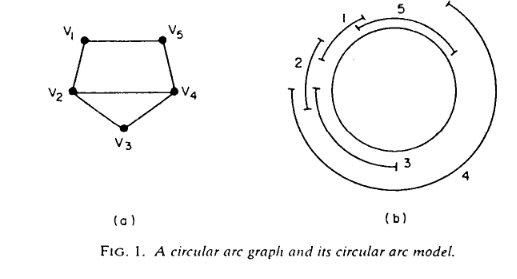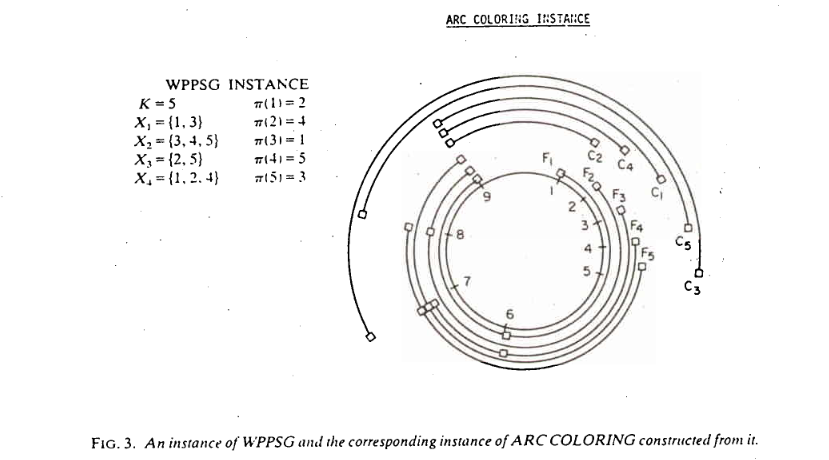# Monthly Archives: September 2021

## Register Sufficiency For Loops

We finally get to the book problem that was the basis for our diversion.

The problem: Register Sufficiency For Loops.   This is problem PO3 in the appendix.

The description: Given a set of variables to be used within a loop of N instructions, and a set of K registers.  Each variable has a “lifetime” defined by the instruction in the loop we start needing its value, and the number of instructions we will that need that value for). We can assign two variables to the same register if they do not overlap in their lifetimes (keeping in mind that “overlap” includes wrapping around to the start of the next loop iteration).  Can we assign variables to registers such that there is no overlap?

Example: I think it’s easier to understand the concept of a “lifetime” of a variable by showing an example of a loop:

B
C
B
C
A
C
A
C
A
C
B
C
B

In this loop, we will need one register to hold C (because we’re basically using it the whole time), but the other register can alternate between holding A and B.  It holds A in the middle of the loop, but it can hold B from the end of one iteration through the start of the next.

If I added an extra B in the middle of the A instructions, I would need 3 registers (because we can’t have 1 register holding A and B because the “middle” use of B would conflict with the need to hold A).

Reduction: While this is the main result for us, this problem is mentioned as an aside in the Garey, Johnson, Miller, and Papadimitriou paper we have been working with for the past few weeks.

The idea is that this problem is very similar to Arc Coloring.  They show the problems are similar, which is sort of like doing the reduction backwards (“Look, any loop can be represented as a set of arcs!”).  But if we start from an Arc Coloring instance, we can create a loop with one statement for each point in the circle, and each arc connects the first and last uses of a variable (so the arc spans the lifetime of the variable).

The idea now is that a coloring of the graph is a way to color the arcs of the graph so that no two arcs overlap at a point.  This is exactly the same as saying we can assign a register to a set of instructions (matching the 2 uses of a variable) so that the same register is not in use during the lifetimes of two variables assigned to that register.

Difficulty: The actual reduction here is a 4.  (I think it’s a little tricky to understand the idea of a lifetime of a variable, and how it can be represented as just 2 points in the circle)  But it may be more complicated than that to explain what an arc graph is, and does.

## Arc Coloring

Last week we did a subproblem along the way to the next problem in the appendix.  This week we are making a second stop.

The problem: Arc Coloring.  This problem is not in the appendix.

The description: Given a circle divided into m equally spaced points, and a set {A1..An} of “arcs”.  Each arc Ai is represented by a pair (ai, bi) signifying an arc that starts at point ai and continues clockwise to point bi

A circular arc graph is a graph built from this set of arcs, with one vertex for each arc, and an edge between two arcs if they intersect at one or more points (the starting point of an interval ai does not count for overlap purposes)

The arc coloring question asks: Given an integer K, and a circular arc graph G, can we color G in K or fewer colors?  Alternately, we are asking whether we can partition the given set of arcs into K or fewer groups with no intersections within any group.

Example: The paper by Garey, Johnson, Miller, and Papadimitriou gives this example of a circular arc graph:The right side is the circle and the arcs, the left side is the graph.  So we can see there are edges connecting v1 to v2 and v5 because arc 1 overlaps with both arcs 2 and 5.

This graph can be colored with 3 colors (v2, v3, and v4 form a clique, so at least 3 colors will be needed, and coloring v1 the same color as v4 and v5 the same color of v1 will color the graph in just 3 colors).

Note that we already know that the general graph coloring problem is NP-Complete.  We are asking here whether this restricted kind of graph can be colored in polynomial time.  It will turn out that coloring this graph will be NP-Complete as well.

Reduction: The reason we talked about the Word Problem for Products of Symmetric Groups last time was to reduce from it here.

So we start with an instance of WPPSG- a collection of sets X1 through Xm, an integer K, and a goal permutation π.  We’ll assume that each number from 1 to K occurs in at least one X set (if not, if π moves that number we have an automatic “no” instance, and if it doesn’t, we can just remove the number from consideration (and shift all larger numbers down 1)).  We want to build a graph that is colorable in K colors or less if and only if our WPPSG instance is solvable.

Our circle will have K+m points on it.  Each set Xi will generate a set of arcs.  Let li be the index of the first X set that contains i, let li be the index of the second such set, and so on.  So using our example from last time, we’d say that l5 is 1 (because X1 is the first set to have a 5 in it), and l5 is 3 (because X3 is the second set to have a 5 in it).  We’ll denote the last such label as li[k(i)]

So, for each Xi we build a set of arcs:

• Ai1 = (i, K+li)
• Ai2 = (K+li, K+li)
• Ai,k(i) = (K+li[k(i)-1], K+li[k(i)])

This creates a set of arcs that are disjoint, and who cover the points from i to K+li[k(i)]

Each set Xi also creates an arc Ci = (K+li[k(i)], π-1(i)). The “π-1(i)” is just the inverse of the π permutation we started with, and tells us for each number from 1..K what position that number should end up in.  This Ci arc continues the Ai arcs from where they “end” and go around to π-1(i).

Here is an example of how this gets built from the paper:The actual proof that this conversion works is hard and complicated.  The general idea is that for each point in the circle, we need a different color for each arc that crosses the point.  The different colors can map to the permutations of the numbers from 1-K.  They show that you can build a permutation of colors that works based on the permutations you can do on the X sets, and so you can get to a legal permutation if and only if you can get to a legal coloring.

Difficulty: 8.  The construction is cool, but hard to see from the initial problem.  The actual proof is very tricky as well.